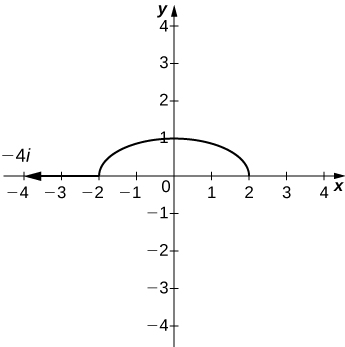$$\newcommand{\id}{\mathrm{id}}$$ $$\newcommand{\Span}{\mathrm{span}}$$ $$\newcommand{\kernel}{\mathrm{null}\,}$$ $$\newcommand{\range}{\mathrm{range}\,}$$ $$\newcommand{\RealPart}{\mathrm{Re}}$$ $$\newcommand{\ImaginaryPart}{\mathrm{Im}}$$ $$\newcommand{\Argument}{\mathrm{Arg}}$$ $$\newcommand{\norm}{\| #1 \|}$$ $$\newcommand{\inner}{\langle #1, #2 \rangle}$$ $$\newcommand{\Span}{\mathrm{span}}$$

# 6.6E:

$$\newcommand{\vecs}{\overset { \rightharpoonup} {\mathbf{#1}} }$$ $$\newcommand{\vecd}{\overset{-\!-\!\rightharpoonup}{\vphantom{a}\smash {#1}}}$$$$\newcommand{\id}{\mathrm{id}}$$ $$\newcommand{\Span}{\mathrm{span}}$$ $$\newcommand{\kernel}{\mathrm{null}\,}$$ $$\newcommand{\range}{\mathrm{range}\,}$$ $$\newcommand{\RealPart}{\mathrm{Re}}$$ $$\newcommand{\ImaginaryPart}{\mathrm{Im}}$$ $$\newcommand{\Argument}{\mathrm{Arg}}$$ $$\newcommand{\norm}{\| #1 \|}$$ $$\newcommand{\inner}{\langle #1, #2 \rangle}$$ $$\newcommand{\Span}{\mathrm{span}}$$ $$\newcommand{\id}{\mathrm{id}}$$ $$\newcommand{\Span}{\mathrm{span}}$$ $$\newcommand{\kernel}{\mathrm{null}\,}$$ $$\newcommand{\range}{\mathrm{range}\,}$$ $$\newcommand{\RealPart}{\mathrm{Re}}$$ $$\newcommand{\ImaginaryPart}{\mathrm{Im}}$$ $$\newcommand{\Argument}{\mathrm{Arg}}$$ $$\newcommand{\norm}{\| #1 \|}$$ $$\newcommand{\inner}{\langle #1, #2 \rangle}$$ $$\newcommand{\Span}{\mathrm{span}}$$

## Directional Derivatives and the Gradient

For the following exercises, find the directional derivative using the limit definition only.

1) $$\displaystyle f(x,y)=5−2x^2−\frac{1}{2}y^2$$ at point $$\displaystyle P(3,4)$$ in the direction of $$\displaystyle u=(cos\frac{π}{4})i+(sin\frac{π}{4})j$$

2) $$\displaystyle f(x,y)=y^2cos(2x)$$ at point $$\displaystyle P(\frac{π}{3},2)$$ in the direction of $$\displaystyle u=(cos\frac{π}{4})i+(sin\frac{π}{4})j$$

Solution:$$\displaystyle −3\sqrt{3}$$

3) Find the directional derivative of $$\displaystyle f(x,y)=y^2sin(2x)$$ at point $$\displaystyle P(\frac{π}{4},2)$$ in the direction of $$\displaystyle u=5i+12j.$$

For the following exercises, find the directional derivative of the function at point P in the direction of v.

4) $$\displaystyle f(x,y)=xy, P(0,−2), v=\frac{1}{2}i+\frac{\sqrt{3}}{2}j$$

Solution:$$\displaystyle −1$$

5) $$\displaystyle h(x,y)=e^xsiny,P(1,\frac{π}{2}),v=−i$$

6) $$\displaystyle h(x,y,z)=xyz,P(2,1,1),v=2i+j−k$$

Solution:$$\displaystyle \frac{2}{\sqrt{6}}$$

7) $$\displaystyle f(x,y)=xy,P(1,1),u=⟨\frac{\sqrt{2}}{2},\frac{\sqrt{2}}{2}⟩$$

8) $$\displaystyle f(x,y)=x^2−y^2,u=⟨\frac{\sqrt{3}}{2},\frac{1}{2}⟩,P(1,0)$$

Solution:$$\displaystyle \sqrt{3}$$

9) $$\displaystyle f(x,y)=3x+4y+7,u=⟨\frac{3}{5},\frac{4}{5}⟩,P(0,\frac{π}{2})$$

10) $$\displaystyle f(x,y)=e^xcosy,u=⟨0,1⟩,P=(0,\frac{π}{2})$$

Solution:$$\displaystyle −1.0$$

11) $$\displaystyle f(x,y)=y^{10},u=⟨0,−1⟩,P=(1,−1)$$

12) $$\displaystyle f(x,y)=ln(x^2+y^2),u=⟨\frac{3}{5},\frac{4}{5}⟩,P(1,2)$$

Solution:$$\displaystyle \frac{22}{25}$$

13) $$\displaystyle f(x,y)=x^2y,P(−5,5),v=3i−4j$$

14) $$\displaystyle f(x,y)=y^2+xz,P(1,2,2),v=⟨2,−1,2⟩$$

Solution:$$\displaystyle \frac{2}{3}$$

For the following exercises, find the directional derivative of the function in the direction of the unit vector $$\displaystyle u=cosθi+sinθj.$$

15) $$\displaystyle f(x,y)=x^2+2y^2,θ=\frac{π}{6}$$

16) $$\displaystyle f(x,y)=\frac{y}{x+2y},θ=−\frac{π}{4}$$

Solution:$$\displaystyle \frac{−\sqrt{2}(x+y)}{2(x+2y)^2}$$

17) $$\displaystyle f(x,y)=cos(3x+y),θ=\frac{π}{4}$$

18) $$\displaystyle w(x,y)=ye^x,θ=\frac{π}{3}$$

Solution:$$\displaystyle \frac{e^x(y+\sqrt{3})}{2}$$

19) $$\displaystyle f(x,y)=xarctan(y),θ=\frac{π}{2}$$

20) $$\displaystyle f(x,y)=ln(x+2y),θ=\frac{π}{3}$$

Solution:$$\displaystyle \frac{1+2\sqrt{3}}{2(x+2y)}$$

For the following exercises, find the gradient.

21) Find the gradient of $$\displaystyle f(x,y)=\frac{14−x^2−y^2}{3}$$. Then, find the gradient at point $$\displaystyle P(1,2).$$

22) Find the gradient of $$\displaystyle f(x,y,z)=xy+yz+xz$$ at point $$\displaystyle P(1,2,3).$$

Solution:$$\displaystyle ⟨5,4,3⟩$$

23) Find the gradient of $$\displaystyle f(x,y,z))$$ at $$\displaystyle P$$ and in the direction of $$\displaystyle u: f(x,y,z)=ln(x^2+2y^2+3z^2),P(2,1,4),u=\frac{−3}{13}i−\frac{4}{13}j−\frac{12}{13}k.$$

24) $$\displaystyle f(x,y,z)=4x^5y^2z^3,P(2,−1,1),u=\frac{1}{3}i+\frac{2}{3}j−\frac{2}{3}k$$

Solution:$$\displaystyle −320$$

For the following exercises, find the directional derivative of the function at point $$\displaystyle P$$ in the direction of $$\displaystyle Q$$.

25) $$\displaystyle f(x,y)=x^2+3y^2,P(1,1),Q(4,5)$$

26) $$\displaystyle f(x,y,z)=\frac{y}{x+z},P(2,1,−1),Q(−1,2,0)$$

Solution:$$\displaystyle \frac{3}{\sqrt{11}}$$

For the following exercises, find the derivative of the function at $$\displaystyle P$$ in the direction of $$\displaystyle u$$.

27) $$\displaystyle f(x,y)=−7x+2y,P(2,−4),u=4i−3j$$

28) $$\displaystyle f(x,y)=ln(5x+4y),P(3,9),u=6i+8j$$

Solution:$$\displaystyle \frac{31}{255}$$

29) [T] Use technology to sketch the level curve of $$\displaystyle f(x,y)=4x−2y+3$$ that passes through $$\displaystyle P(1,2)$$ and draw the gradient vector at $$\displaystyle P$$.

30) [T] Use technology to sketch the level curve of $$\displaystyle f(x,y)=x^2+4y^2$$ that passes through $$\displaystyle P(−2,0)$$ and draw the gradient vector at P.

Solution:For the following exercises, find the gradient vector at the indicated point.

31) $$\displaystyle f(x,y)=xy^2−yx^2,P(−1,1)$$

32) $$\displaystyle f(x,y)=xe^y−ln(x),P(−3,0)$$

Solution:$$\displaystyle \frac{4}{3}i−3j$$

33) $$\displaystyle f(x,y,z)=xy−ln(z),P(2,−2,2)$$

34) $$\displaystyle f(x,y,z)=x\sqrt{y^2+z^2}, P(−2,−1,−1)$$

Solution:$$\displaystyle \sqrt{2}i+\sqrt{2}j+\sqrt{2}k$$

For the following exercises, find the derivative of the function.

35) $$\displaystyle f(x,y)=x^2+xy+y^2$$ at point $$\displaystyle (−5,−4)$$ in the direction the function increases most rapidly

36) $$\displaystyle f(x,y)=e^{xy}$$ at point $$\displaystyle (6,7)$$ in the direction the function increases most rapidly

Solution:$$\displaystyle 1.6(10^{19})$$

37) $$\displaystyle f(x,y)=arctan(\frac{y}{x})$$ at point $$\displaystyle (−9,9)$$ in the direction the function increases most rapidly

38) $$\displaystyle f(x,y,z)=ln(xy+yz+zx)$$ at point $$\displaystyle (−9,−18,−27)$$ in the direction the function increases most rapidly

Solution:$$\displaystyle \frac{5\sqrt{2}}{99}$$

39) $$\displaystyle f(x,y,z)=\frac{x}{y}+\frac{y}{z}+\frac{z}{x}$$ at point $$\displaystyle (5,−5,5)$$ in the direction the function increases most rapidly

For the following exercises, find the maximum rate of change of $$\displaystyle f$$ at the given point and the direction in which it occurs.

40) $$\displaystyle f(x,y)=xe^{−y}, (1,0)$$

Solution:$$\displaystyle \sqrt{5},⟨1,2⟩$$

41) $$\displaystyle f(x,y)=\sqrt{x^2+2y}, (4,10)$$

42) $$\displaystyle f(x,y)=cos(3x+2y),(\frac{π}{6},−\frac{π}{8})$$

Solution:$$\displaystyle \sqrt{\frac{13}{2}},⟨−3,−2⟩$$

For the following exercises, find equations of

a. the tangent plane and

b. the normal line to the given surface at the given point.

43) The level curve $$\displaystyle f(x,y,z)=12$$ for $$\displaystyle f(x,y,z)=4x^2−2y^2+z^2$$ at point $$\displaystyle (2,2,2).$$

44) $$\displaystyle f(x,y,z)=xy+yz+xz=3$$ at point $$\displaystyle (1,1,1)$$

Solution:$$\displaystyle a. x+y+z=3, b. x−1=y−1=z−1$$

45) $$\displaystyle f(x,y,z)=xyz=6$$ at point $$\displaystyle (1,2,3)$$

46) $$\displaystyle f(x,y,z)=xe^ycosz−z=1$$ at point $$\displaystyle (1,0,0)$$

Solution:$$\displaystyle a. x+y−z=1, b. x−1=y=−z$$

For the following exercises, solve the problem.

47) The temperature $$\displaystyle T$$ in a metal sphere is inversely proportional to the distance from the center of the sphere (the origin: $$\displaystyle (0,0,0))$$. The temperature at point $$\displaystyle (1,2,2)$$ is $$\displaystyle 120°C.$$

a. Find the rate of change of the temperature at point $$\displaystyle (1,2,2)$$ in the direction toward point $$\displaystyle (2,1,3).$$

b. Show that, at any point in the sphere, the direction of greatest increase in temperature is given by a vector that points toward the origin.

48) The electrical potential (voltage) in a certain region of space is given by the function $$\displaystyle V(x,y,z)=5x^2−3xy+xyz.$$

a. Find the rate of change of the voltage at point $$\displaystyle (3,4,5)$$ in the direction of the vector $$\displaystyle ⟨1,1,−1⟩.$$

b. In which direction does the voltage change most rapidly at point $$\displaystyle (3,4,5)$$?

c. What is the maximum rate of change of the voltage at point $$\displaystyle (3,4,5)$$?

Solution:$$\displaystyle a. \frac{32}{\sqrt{3}}, b. ⟨38,6,12⟩, c. 2\sqrt{406}$$

49) If the electric potential at a point $$\displaystyle (x,y)$$ in the xy-plane is $$\displaystyle V(x,y)=e^{−2x}cos(2y)$$, then the electric intensity vector at $$\displaystyle (x,y)$$ is $$\displaystyle E=−∇V(x,y).$$

a. Find the electric intensity vector at $$\displaystyle (\frac{π}{4},0).$$

b. Show that, at each point in the plane, the electric potential decreases most rapidly in the direction of the vector $$\displaystyle E.$$

50) In two dimensions, the motion of an ideal fluid is governed by a velocity potential $$\displaystyle φ$$. The velocity components of the fluid u in the x-direction and v in the y-direction, are given by $$\displaystyle ⟨u,v⟩=∇φ$$. Find the velocity components associated with the velocity potential $$\displaystyle φ(x,y)=sinπxsin2πy.$$

Solution:$$\displaystyle ⟨u,v⟩=⟨πcos(πx)sin(2πy),2πsin(πx)cos(2πy)⟩$$